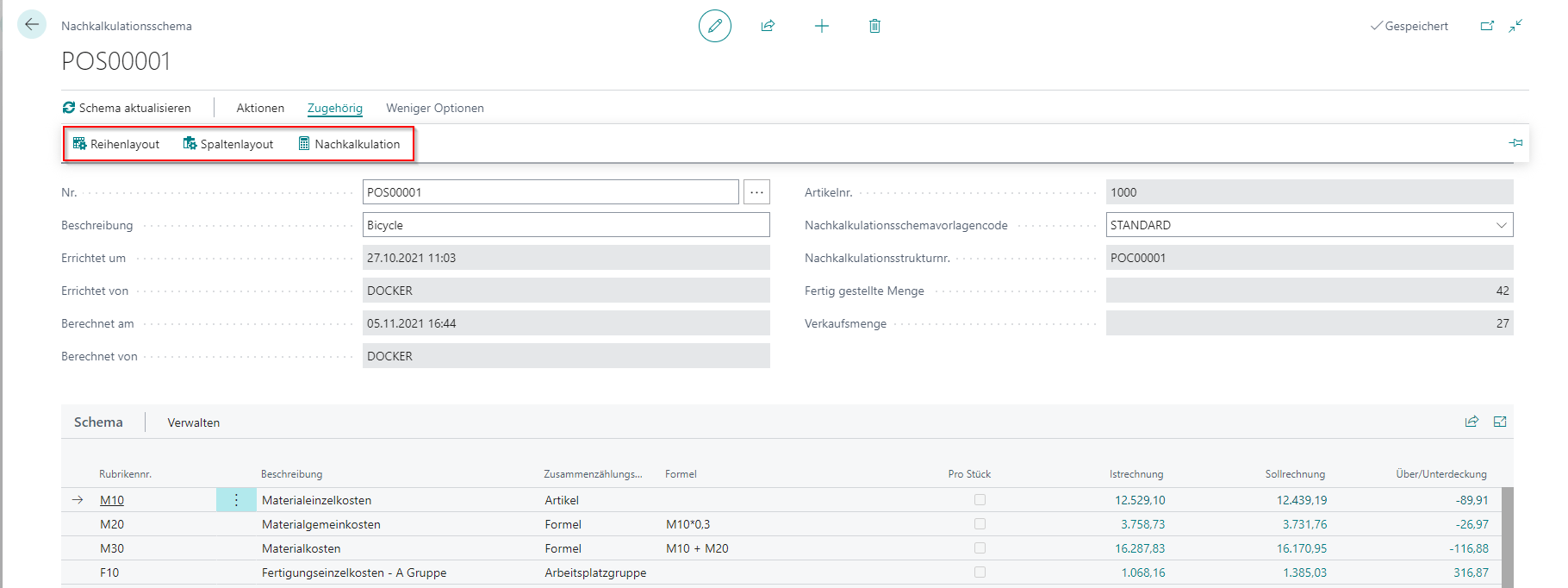# SchemasThe post calculation schemes are for the evaluation of the individual calculations.
If you do not have a post calculation schema template stored, you can also enter the schemas manually.The row layout and column layout is similar to the logic in the schema templates.
If you change anything in the scheme, you need to update the scheme. After that, the corresponding entries will be recalculated.

### Calculation Entries and Calculation Values

Calculation Entries

When updating the scheme, so-called calculation entries are created. These form the basis for further evaluation.For item, machine center, work center the individual lines of the calculation are transferred. At this point, the filters of the schema are also taken into account. If, for example, only a certain item category is evaluated, only the corresponding items appear in the items for this line of the schema. The individual surcharges are also calculated here, if they are required.

You can open the entries via the lookup by clicking on the number of a line with the appropriate summation type.

Calculation Value

The calculation values are the “results” of the calculation. For each combination of row (line) and column, the associated value is logged there and displayed in the page.